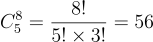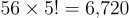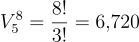02 Oct 2023

Posted on:

01 Oct 2023

0

# Resolved:Combinatorics Homework, Ex 2, answers C and D.

For answers C, D, please confirm if my explanations for both of them are correct:

c) We need to select 5 out of the 8 banners to use. We use different ones for each platform, so repetition is not allowed.
The answer provided uses the formula for combinations without repetition, which would be accurate if the problem were about selecting 5 out of 8 banners without concern for their order. However, the original problem is about arranging these selected banners across 5 different platforms, where the order matters. Therefore, permutations should be used, not combinations. The correct number of options should be 6720, not 56.

d) Now, we need to select 5 out of the 8 banners to use. However, we can choose some multiple times.
The answer here uses the formula for combinations with repetition. Again, this would be correct if the question were solely about selecting banners. However, the problem's constraints specify that these banners will be arranged across 5 different platforms. In such cases, the order matters, and variations with repetition should be used, not combinations with repetition. The correct number of options would be 85=3276885=32768, not 792.

Instructor
Posted on:

02 Oct 2023

0

Hey Youssef,

Thank you for reaching out!

Regarding question 2c, I agree that there can be two ways to read the task, both of which are valid given the way the question is phrased. Let's study the following result:This is the number of unique sets, containing 5 banners each, that we can form from 8 different banners. This result does not consider for the distribution of these banners accross the different platforms. If we want to take this into consideration, we need to multiply this result by the number of ways in which we can arrange all 5 platforms. That would be:This is equivalent to finding the variations of 5 elements from 8 distinct elements.Regarding question 2d, the discrepancy between your answer and the lecturer's ansewer stems from the same reasoning.

Hope this helps!

Kind regards,

365 Hristina